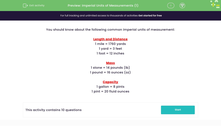# Understand Imperial Units of Measurement

In this worksheet, students will look at how imperial units of measurement work.Key stage:  KS 3

Curriculum topic:   Number

Curriculum subtopic:   Use Standard Units of Measure

Difficulty level:#### Worksheet Overview

We need to know about the following common imperial units of measurement:

Length and Distance

1 mile = 1,760 yards

1 yard = 3 feet

1 foot = 12 inches

Mass

1 stone = 14 pounds (lb)

1 pound = 16 ounces (oz)

Capacity

1 gallon = 8 pints

1 pint = 20 fluid ounces

You might be asked to use this information to change between different forms of measurement.

For example, you might be asked how many pints of water it will take to fill three gallon buckets.To answer this question, we need to look to see how many pints there are in one gallon.

1 gallon = 8 pints

3 gallons = 8 x 3 = 24

So, there are 24 pints in 3 gallons.

Let's try some questions now.

### What is EdPlace?

We're your National Curriculum aligned online education content provider helping each child succeed in English, maths and science from year 1 to GCSE. With an EdPlace account you’ll be able to track and measure progress, helping each child achieve their best. We build confidence and attainment by personalising each child’s learning at a level that suits them.

Get started